# Everett interpolation formula

(diff) ← Older revision | Latest revision (diff) | Newer revision → (diff)

A method of writing the interpolation polynomial obtained from the Gauss interpolation formula for forward interpolation at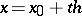with respect to the nodes, that is,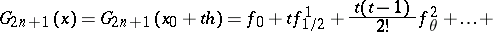without finite differences of odd order, which are eliminated by means of the relation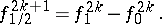Adding like terms yields Everett's interpolation formula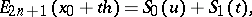(1)

where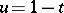and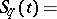(2)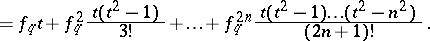Compared with other versions of the interpolation polynomial, formula (1) reduces approximately by half the amount of work required to solve the problem of table condensation; for example, when a given table of the values of a function atis to be used to draw up a table of the values of the same function at,, whereis an integer, the valuesfor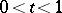are computed be means of the formula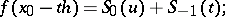andis used to find both values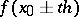.

In manual calculation, in the caseit is advisable to approximate the coefficient of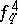in (2) by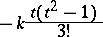and instead ofto compute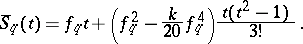The parametercan be chosen, for example, from the condition that the principal part of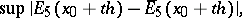where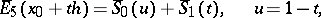has a minimum value. In this case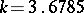.

How to Cite This Entry:
Everett interpolation formula. Encyclopedia of Mathematics. URL: http://encyclopediaofmath.org/index.php?title=Everett_interpolation_formula&oldid=18723
This article was adapted from an original article by M.K. Samarin (originator), which appeared in Encyclopedia of Mathematics - ISBN 1402006098. See original article